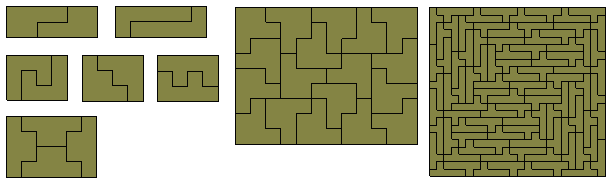Rectifiable PolyominoesA polyomino is said to be 'rectifiable' if a certain number of copies of the polyomino will together form a rectangle. The diagram below shows a tromino and two tetrominoes forming rectangles. It is easy to show that these are the only trominoes and tetrominoes for which this is possible.The tromino above forms a rectangle with two pieces but the same tromino can form different rectangles. For example below 21 copies form a 9x7 rectangle.These two diagrams illustrate the first two definitions below related to rectifiability.

 1 The order of a polyomino is the smallest number of pieces needed to form a rectangle. 2 A rectifiable polyomino is said to be odd if an odd number of pieces can be used to form a rectangle. 3 A rectangle formed with congruent polyominoes is said to be prime if it cannot be formed from smaller rectangles.

There are three pentominoes which are rectifiable. Notice that here we have a pentomino of order 10. All of these pentominoes are also odd.There are eight rectifiable hexominoes (see below). The Y-hexomino is rectifiable and is of order 92 in a 23x24 rectangle!To see lists of prime boxes and drawings of many polyominoes see Erich Friedman's Polyominoes in rectangles (this has most of the diagrams but is missing some primes) or Michael Reid's Rectifiable polyomino page (fewer diagrams but more primes). Also see lists of possible rectangles and Karl Dahlke's Tiling Rectangles With Polyominoes

Irrectifiable Polyominoes

Karl Scherer has introduced the idea of irrectifibility which is the tiling of a rectangle with a set of similar pieces. The examples here use polyominoes which themselves are not rectifiable. Little is known about this type of construction.Rectifiable Pairs of Polyominoes

For the polyomiones which are not rectifiable there are certain pairs which are mutually rectifiable in the sense that copies of the two can together form rectangles. Click on one of the links below for more detail.